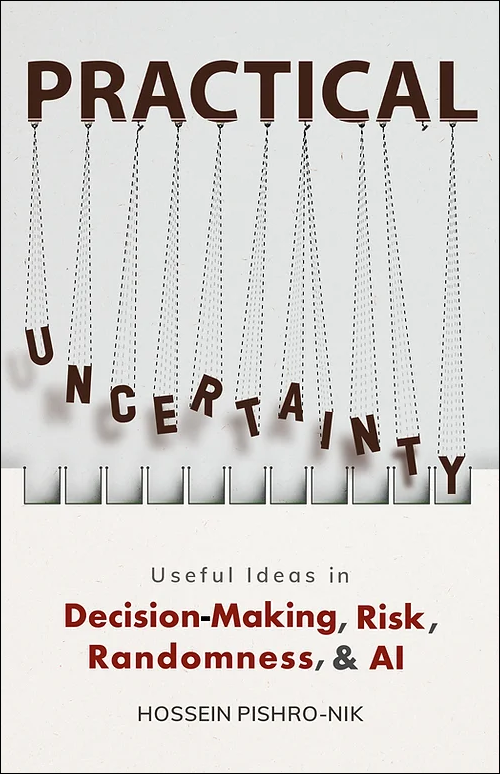## 4.3.3 Solved Problems:Mixed Random Variables

Problem

Here is one way to think about a mixed random variable. Suppose that we have a discrete random variable $X_d$ with (generalized) PDF and CDF $f_d(x)$ and $F_d(x)$, and a continuous random variable $X_c$ with PDF and CDF $f_{c}(x)$ and $F_c(x)$. Now we create a new random variable $X$ in the following way. We have a coin with $P(H)=p$. We toss the coin once. If it lands heads, then the value of $X$ is determined according to the probability distribution of $X_d$. If the coin lands tails, the value of $X$ is determined according to the probability distribution of $X_c$.

1. Find the CDF of $X, F_X(x)$.
2. Find the PDF of $X, f_X(x)$.
3. Find $EX$.
4. Find Var$(X)$.

• Solution
1. Find the CDF of $X, F_X(x)$: We can write  $F_X(x)$ $=P(X \leq x)$ $=P(X \leq x |H) P(H)+P(X \leq x |T) P(T) \textrm{(law of total probability)}$ $=p P(X_d \leq x)+(1-p)P(X_c \leq x)$ $=p F_d(x)+(1-p)F_c(x)$.

2. Find the PDF of $X, f_X(x)$: By differentiating $F_X(x)$, we obtain  $f_X(x)$ $=\frac{dF_X(x)}{dx}$ $=p f_d(x)+(1-p)f_c(x)$.

3. Find $EX$: We have  $EX$ $=\int_{-\infty}^{\infty} xf_X(x)dx$ $=p \int_{-\infty}^{\infty} xf_d(x)dx+(1-p)\int_{-\infty}^{\infty} xf_c(x)dx$ $=pEX_d+(1-p)EX_c$.

4. Find Var$(X)$:  $EX^2$ $=\int_{-\infty}^{\infty} x^2f_X(x)dx$ $=p \int_{-\infty}^{\infty} x^2f_d(x)dx+(1-p)\int_{-\infty}^{\infty} x^2f_c(x)dx$ $=pEX^2_d+(1-p)EX^2_c$.

Thus,  $\textrm{Var}(X)$ $=EX^2-(EX)^2$ $=pEX^2_d+(1-p)EX^2_c- (pEX_d+(1-p)EX_c)^2$ $=pEX^2_d+(1-p)EX^2_c-p^2(EX_d)^2-(1-p)^2 (EX_c)^2-2p(1-p)EX_d EX_c$ $=p(EX^2_d-(EX_d)^2)+(1-p)(EX^2_c-(EX_c)^2)+p(1-p)(EX_d-EX_c)^2$ $=p\textrm{Var}(X_d)+(1-p)\textrm{Var}(X_c)+p(1-p)\big(EX_d-EX_c\big)^2$.

Problem

Let $X$ be a random variable with CDF \begin{equation} \nonumber F_X(x) = \left\{ \begin{array}{l l} 1 & \quad x \geq 1 \\ \frac{1}{2}+\frac{x}{2} & \quad 0 \leq x < 1 \\ 0 & \quad x < 0 \end{array} \right. \end{equation}

1. What kind of random variable is X: discrete, continuous, or mixed?
2. Find the PDF of X, $f_X (x)$.
3. Find $E(e^X)$.
4. Find $P(X=0|X \leq 0.5)$.

• Solution
1. What kind of random variable is X: discrete, continuous, or mixed? We note that the CDF has a discontinuity at $x=0$, and it is continuous at other points. Since $F_X(x)$ is not flat in other locations, we conclude $X$ is a mixed random variable. Indeed, we can write $$F_X(x)=\frac{1}{2} u(x)+ \frac{1}{2} F_Y(x),$$ where $Y$ is a $Uniform(0,1)$ random variable. If we use the interpretation of Problem 1, we can say the following. We toss a fair coin. If it lands heads then $X=0$, otherwise $X$ is obtained according the a $Uniform(0,1)$ distribution.
2. Find the PDF of X, $f_X (x)$: By differentiating the CDF, we obtain $$f_X(x)=\frac{1}{2} \delta(x)+ \frac{1}{2} f_Y(x),$$ where $f_Y(x)$ is the PDF of $Uniform(0,1)$, i.e., \begin{equation} \nonumber f_Y(x) = \left\{ \begin{array}{l l} 1 & \quad 0 < x < 1 \\ 0 & \quad \text{otherwise} \end{array} \right. \end{equation}
3. Find $E(e^X)$: We can use LOTUS to write  $E(e^X)$ $=\int_{-\infty}^{\infty} e^xf_X(x)dx$ $=\frac{1}{2} \int_{-\infty}^{\infty} e^x \delta(x)dx+ \frac{1}{2} \int_{-\infty}^{\infty} e^x f_Y(x)dx$ $=\frac{1}{2} e^0+\frac{1}{2} \int_{0}^{1} e^x dx$ $=\frac{1}{2}+\frac{1}{2}(e-1)$ $=\frac{1}{2}e$.

Here is another way to think about this part: similar to part (c) of Problem 1, we can write  $E(e^X)$ $=\frac{1}{2} \times e^{0} + \frac{1}{2} E[e^Y]$ $=\frac{1}{2} +\frac{1}{2} \int_{0}^{1} e^y dy$ $=\frac{1}{2}e$.

4. Find $P(X=0|X \leq 0.5)$: We have  $P(X=0|X \leq 0.5)$ $=\frac{P(X=0, X \leq 0.5)}{P(X \leq 0.5)}$ $=\frac{P(X=0)}{P(X \leq 0.5)}$ $=\frac{0.5}{\int_{0}^{0.5}f_X(x)dx}$ $=\frac{0.5}{0.75}=\frac{2}{3}$.

Problem

Let $X$ be a $Uniform(-2,2)$ continuous random variable. We define $Y=g(X)$, where the function $g(x)$ is defined as \begin{equation} \nonumber g(x)=\left\{ \begin{array}{l l} 1 & \quad x > 1 \\ x & \quad 0 \leq x \leq 1\\ 0 & \quad \text{otherwise} \end{array} \right. \end{equation} Find the CDF and PDF of $Y$.

• Solution
• Note that $R_Y=[0,1]$. Therefore, $$F_Y(y)=0, \quad \textrm{for} \quad y<0,$$ $$F_Y(y)=1, \quad \textrm{for} \quad y \geq 1.$$ We also note that $$P(Y=0)=P(X < 0)=\frac{1}{2},$$ $$P(Y=1)=P(X > 1)=\frac{1}{4}.$$ Also for $0 < y < 1$, $$F_Y(y)=P(Y \leq y)= P(X \leq y)=F_X(y)=\frac{y+2}{4}.$$ Thus, the CDF of $Y$ is given by \begin{equation} \nonumber F_Y(y)=\left\{ \begin{array}{l l} 1 & \quad y \geq 1 \\ \frac{y+2}{4} & \quad 0 \leq y < 1\\ 0 & \quad \text{otherwise} \end{array} \right. \end{equation} In particular, we note that there are two jumps in the CDF, one at $y=0$ and another at $y=1$. We can find the generalized PDF of $Y$ by differentiating $F_Y(y)$: $$f_Y(y)=\frac{1}{2} \delta(y)+\frac{1}{4} \delta(y-1)+\frac{1}{4} (u(y)-u(y-1)).$$

 The print version of the book is available on Amazon.Practical uncertainty: Userful Ideas in Decision-Making, Risk, Randomness, & AI